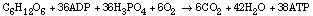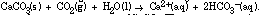## Within Chemistry

1. Since the mole is the central concept in quantitative chemistry, it is related to almost all topics supported in high school chemistry. Here are some examples:

a.Chemical Reactions and Stoichiometry . The law of conservation of mass requires that the same numbers of atoms of all species be present before and after reaction. Because the small size of atoms makes it impractical to count atoms, chemists use moles of atoms. In balancing chemical equations, the coefficients are related to the moles of substances present. The relationship of moles of reactants and products in balanced equations is central in determining mass relationships in chemical reactions (stoichiometry).

b.Solutions. Chemists generally express solution concentration in terms of molarity (moles of solute per liter of solution) or mole fraction (moles of solute per total moles in solution). Because chemical reactions are based on moles, molarity is convenient in determining the quantities of solutions needed for chemical reactions. Colligative properties of solutions (freezing point depression, boiling point elevation, osmotic pressure, vapor pressure lowering) are related to the moles of solute particles in solution.

c.Rates of Reaction . Rates of reaction are often expressed in terms of moles of reactant per liter (mol/L) disappearing per unit time (or some quantity related to molarity, such as pressure of gas, absorbance/transmittance of light, etc.).

d.Equilibrium. Since coefficients in a balanced equation relate moles of reactants and products, quantities of substances present at equilibrium are usually expressed in terms of molarity (moles/liter) for reactions in solution or pressure (which is proportional to moles per liter) for gases.

e. Acids and Bases. A monoprotic acid is a compound that can furnish one mole of hydrogen (hydronium) ions per mole of compound. A diprotic acid can furnish two moles of hydrogen ions per mole of compound; a triprotic acid three. When soluble acids and bases react, the net ionic equation involves one mole of hydrogen (hydronium) ion and one mole of hydroxide ion. The pH of a solution is related to the molarity (moles/liter) of hydrogen (hydronium) ion present in solution.

f. Electrochemistry and Oxidation-Reduction . In oxidation-reduction reactions, transfer of electrons accompanies chemical change. Electrochemical reactions can be described by indicating the number of moles of electrons (Faradays) transferred per mole of reactant species oxidized or reduced. In electrolysis reactions, current, time, and amount of product are related to the moles of electrons involved.

g. Gases. The amount (moles) of a gas sample as well as its temperature and pressure determine its volume. (The ideal gas law relates these four quantities.) Since the volume of a gas at constant temperature and pressure is proportional to the amount of gaseous substance, the relative volumes of reactant and product gases provide a convenient method for determining or monitoring the stoichiometry of gaseous reactions.

h. Thermochemistry . In a thermochemical equation, the physical states of all reactants and products as well as the mole relationships among reactants and products determine the quantity of energy involved. The thermal energy involved in a reaction is proportional to the amounts (moles) of reactants used and products formed.

i. Condensed States. The molar heat of fusion (thermal energy required to melt one mole of substance), the molar heat of vaporization (thermal energy required to evaporate one mole of substance), and the molar heat capacity (thermal energy required to raise the temperature of one mole of substance by one degree) are all commonly used quantities.

2. When chemists prepare new compounds (particularly organic compounds), one way the compound can be characterized is from the elemental analysis. A sample of the compound is sent to a laboratory that provides the mass percent of each element (e.g., % C, % H, % O, % N, etc.). From this information, the chemist can derive the empirical formula for the compound. Generally, spectral data (infrared, ultraviolet, nuclear magnetic resonance, mass spectrum, etc.) are used to predict the structure and to deduce the arrangement of the atoms in the molecule. From spectral data and knowledge of chemistry (prediction of the reaction products based on analogous reactions with known products), the chemist proposes a formula and structure for the compound. If the empirical formula of the proposed compound and the empirical formula predicted from elemental analysis agree, the proposed structure is possible. If they do not agree, the proposed structure is incorrect and additional studies must be made to identify the compound.

## Between Chemisty and Other Disciplines

Since the mole is central to chemistry, related areas such as medicine, biochemistry, biology, geology, physics, agronomy, and environmental science use the mole concept with reference to chemical processes important in those disciplines. A few examples are given below.

1. The mole (particularly millimoles and molarity) is a commonly used unit in medicine. For example, concentrations of intravenous solutions and drugs are expressed in terms of moles.

2. For the complete oxidation of glucose using the glycolytic pathway and the Krebs cycle, 38 moles of ATP are produced per mole of glucose metabolized.The amount of ATP formed in a biochemical reaction is related to the energy since the hydrolysis of each mole of ATP corresponds to 31 kJ of free energy. For the metabolism of glucose in the Krebs cycle, energy production efficiency is roughly 40% (approximately 1.2 x 103 kJ/mol glucose in the Krebs cycle compared to approximately 2.9 x 103kJ/mol glucose for combustion of glucose to CO2and H2O). This is higher efficiency than in the automobile engine (about 20%) and most other heat engines (maximum efficiency about 35%).

3. Formation of stalactites and stalagmites involves the dissolution and reprecipitation of calcium carbonate. Dissolved CO2 causes limestone (CaCO 3 ) to dissolve according to the reactionThe reverse reaction results in reprecipitation of CaCO 3 . The concentration (moles/ liter) of dissolved C 2in water seeping through limestone caves is one factor responsible for formation of stalactites and stalagmites.

4. One source of "fixed" nitrogen (nitrogen that can be used by plants) is the combination of nitrogen and oxygen during highly energetic conditions such as during a lightning storm. The reaction is; 2 NO(g). The NO is further oxidized to NO2 , which dissolves in water to form nitric and nitrous acids.The quantities of nitrogen "fixed" is related to the moles of nitrogen reacting with oxygen.

## To The Contemporary World

Community

Knowledgable Individuals

A pharmacist or clinical laboratory scientist (medical technologist) could explain how the mole concept is used in medicine.

Societal

1. Commercially available materials sometimes list the percent composition, particularly with respect to important or active components in the formulation. For example, the label on cleaning agents (such as dishwasher detergent) may include the percent phosphorus (by mass).

2. The composition of soil fertilizers is generally expressed in terms of % P 2O5 (or H3 PO4 ), % N (or NH3 ) and % K2O (see module on Industrial Inorganic Chemistry).

3. The relationship of moles to chemical reactions opens an inexhaustible supply of applications such as combustion of gasoline in automobile engines, reaction of SO2(from the burning of sulfur-containing fossil fuels) with CaCO3 (marble, limestone, etc. in statuary and building materials), as well as the production of "acid rain" from SO2 . (For additional examples see module on Stoichiometry.)